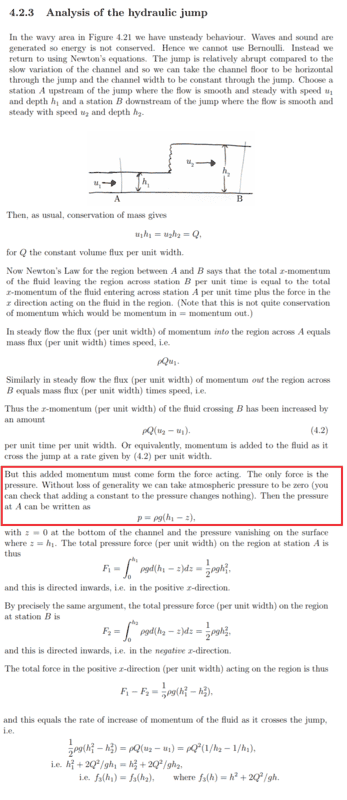# Question about hydraulic jump assumptions

Clara ChungSorry for the poor image... A better picture can be viewed here https://www.dropbox.com/s/uyi01l27vu2fwyw/96.png?dl=0
I don't understand the assumption in the red box...
If I don't ignore the atmospheric pressure, F_1 = integrate from 0 to h_1 (ρg(h_1-z)+p_a)dz = 1/2 ρgh_1^2 + p_a*h_1, similarly for F_2.. Therefore, the final line would be f_3(h) = h^2 + 2Q^2/gh +2/ρg*p_a*h which a linear term is added...

Homework Helper
Gold Member
•Clara Chung
swara31
••